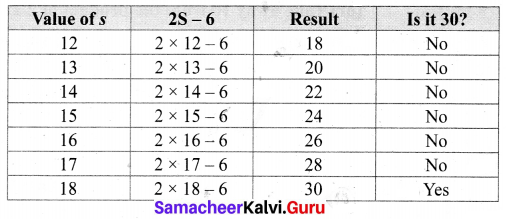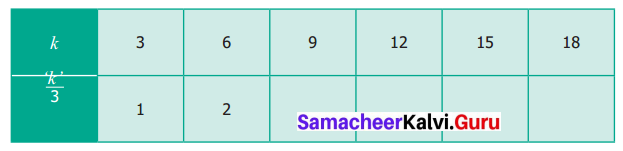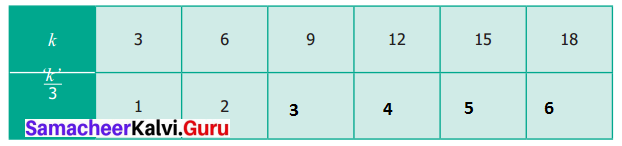# Samacheer Kalvi 6th Maths Solutions Term 1 Chapter 2 Introduction to Algebra Ex 2.2

You can Download Samacheer Kalvi 6th Maths Book Solutions Guide Pdf, Tamilnadu State Board help you to revise the complete Syllabus and score more marks in your examinations.

## Tamilnadu Samacheer Kalvi 6th Maths Solutions Term 1 Chapter 2 Introduction to Algebra Ex 2.2Question 1.
Find in the blanks.
(i) The algebraic statement of ‘f’ decreased by 5 is _______
(ii) The algebraic statement of ‘s’ divided by 5 is _______
(iii) The verbal statement of ‘2m – 10’ is _______
(iv) If A’s age is ‘n’ years now, 7 years ago A’s age was ______
(v) If ‘p – 5’ gives 12 then ‘p’ is ______
Solution:
(i) f – 5
(ii) $$\frac { s }{ 5 }$$
(iii) 10 less than 2 times m (or) Take away 10 from the product of 2 and m
(iv) n – 7
(v) 17
Hint: n – 5 = 12⇒ n = 12 + 5 = 17

Question 2.
Say True or False.
(i) 10 more to three times ‘c’ is ‘3c + 13’.
(ii) If the cost of 10 rice bags is Rs ‘t’, then the cost of 1 rice bag is Rs $$\frac{t}{10}$$
(iii) The statements ‘x’ divided by 3 and 3 divided by ‘x’ are the same.
(iv) The product of ‘q’ and 20 is ‘20q’.
(v) 7 less to 7 times ‘y’ is ‘7 – 7y’.
Solution:
(i) False
(ii) True
(iii) False
(iv) True
(v) False

Question 3.
Express the following verbal statement to algebraic statement.
(i) ‘t’ is added to 100
(ii) 4 times ‘q’
(iii) 8 is reduced by ‘y’
(iv) 56 added to 2 times ‘x’
(v) 4 less to 9 times of ‘y’
Solution:
(i) t + 100
(ii) 4q
(iii) 8 – y
(iv) 2x + 56
(v) 9y – 4Question 4.
Express the following algebraic statement to verbal statement.
(i) x ÷ 3
(ii) 5n – 12
(iii) 11 + 10x
(iv) 70s
Solution:
(i) x divided by 3
(ii) 12 less to 5 times n
(iii) 11 added to 10 times x
(iv) 70 times s

Question 5.
The teacher asked two students to write the algebraic statement for the verbal statement “8 more than a number” on the board. Vetri wrote ‘8 + x’ but Maran wrote ‘8x’. Who gave the correct answer?
Solution:
Let the number be x; 8 more than the number = 8 + x.
Vetri gave the correct answer as 8 + x.

Question 6.
Answer the following questions:
(i) If ‘n’ takes the value 3 then find the value of ‘n + 10’
(ii) If ‘g’ is equal to 300. What is the value of ‘g – 1’ and ‘g + 1’?
(iii) What is the value of s; if ‘2s – 6’ gives 30?
Solution:
(i) Given n = 3
then n + 10 = 3 + 10 = 13
n + 10 = 13

(ii) Given g = 300
g – 1 = 300 – 1 = 299
g + 1 = 300 + 1 = 301
g – 1 = 299; g + 1 = 301

(iii) Given 2s – 6 = 30
From the table:
2 × 18 – 6 = 30
s = 18Question 7.
Complete the table and find the value of ‘k’ for which $$\frac { k }{ 3 }$$ gives 5.Solution:
Given: k ÷ 3 = 5 From the above table we find that
15 ÷ 3 = 5
k = 5Objective Type Questions

Question 8.
The value of ‘y’ in y + 7 = 13 is
(a) y = 5
(b) y = 6
(c) y = 7
(d) y = 8
Solution:
(b) y = 6

Question 9.
6 less to ‘n’ gives 8 is represented as
(a) n – 6 = 8
(b) 6 – n = 8
(c) 8 – n = 6
(d) n – 8 = 6
Solution:
(a) n – 6 = 8

Question 10.
The value of ‘c’ for which $$\frac{3c}{4}$$ gives 18 is
(a) c = 15
(b) c = 21
(c) c = 24
(d) c = 27
Solution:
(c) c = 24